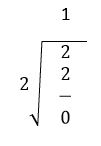# Binary number system

• Post author:
• Post category:DLD

# Binary number system

A number which expressed base-2 is known as binary numbers.

For example: 910 = 10012
In computer system the transformer, semiconductors and wires etc are use to handle the information and all these are only understand the two states: on (state 1) or off (state 0). In binary number system we use only two symbols or digit: zero (0) or one (1) to represent the absence or presence of information.

 Binary state On (1) Off (0) Bulb 1 0 Switch 1 0 Circuit pulse 1 0

#### Binary arithmetic

Using binary numbers computer can perform four functions. Binary arithmetic is simpler to understand because binary numbers use only two states: zero (0) or one (1). To performing arithmetic function in binary number the answer will be in 0s or 1s.

Functions

#Binary subtraction
#Binary multiplication
#Binary division

0+0=0
0+1=1
1+0=1
1+1=0

(1 is carry to next higher column)

Method of solving the number greater than 1:If the number is greater than 1 than it solves using division method. The reminder is use as result of binary addition and the quotient is used as carry to next higher column.

Example:

add binary numbers 111+101 in binary forms.Example

add binary numbers 101=110 in binary forms.#### Binary subtraction

In binary subtraction we follow two steps; the first step is, if the lower digit is larger than the upper digit than it is necessary to borrow from the column to the left. It is very important to know that the borrowed value depends upon the base of the number system and the number is always the decimal equivalent to the base. The second step is, simply subtracting the lower value to the larger value.

Subtraction table

0-0=0

0-1=1 (with a borrow from the next column)

1-0=1

1-1=0

ExampleExample

Subtract 011102 from 101012In this example firstly we subtract 0 from 1 the result is 1. In second step we subtract from 1 from 0 it is not possible than we require borrow from third column than we subtract 1 from 2 than the result is 1. In third step we subtract 1 from 0 due to previous borrow than borrow is require we contain borrow from fourth column it is not possible because the value is 0 than we contain borrow from fifth column now subtract 1 from 2 than the result is 1. In fourth step we subtract 1 from 1 the result is 0. In fifth step subtract 0 from 0 the result is 0.

#### Binary multiplication

Binary multiplication is very simple we cannot require any complex method to multiply binary numbers.

Multiplication table

0 x 0 = 0
0 x 1 = 0
1 x 0 = 0
1 x 1 = 1

Example

Multiply binary numbers 1010 and 1001Example

Multiply binary numbers 1111 and 111#### The rule of binary division is

1) Started from the left side to the dividend.
2) Perform a series of subtraction.
3) If subtraction is possible than put 1 in the quotient and subtract the divisor
from the corresponding digits of dividend.
4) If subtraction is not possible record 0 in the quotient.
5) Bring down the next digit to the remainder digits.

Division table

0/0= divided by zero error
0/1=0
1/0= divided by zero error
1/1=1
Example

Divided 1000012 by 1102The result is dividing 3310 (1000012) by 610 (1102) gives quotient of 510 (1012) and remainder is 310 (112)

DLD (Digital Logic Design) Introduction